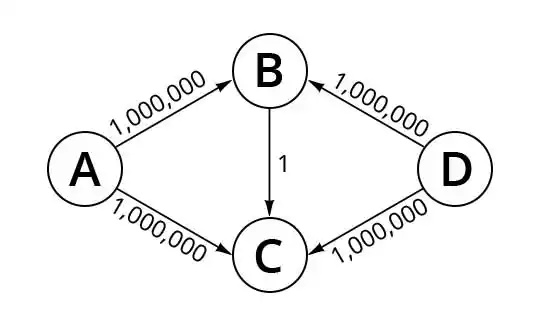### Worst-Case Complexity of Ford-Fulkerson

Ask a computer scientist the worst-case complexity of the Ford-Fulkerson algorithm1 with integral edge capacities, and you'll likely get the answer of O(E*|f|), where E is the number of edges in the graph, and |f| is the capacity of the maximum flow, f, that the algorithm seeks to find. Indeed, that would have been my answer, as well. Until I tried to exploit that worst-case behavior.

Recently, I have been developing Java programs containing algorithmic complexity vulnerabilities, in order to test vulnerability detection tools being developed. Ford-Fulkerson seemed a perfect example of an algorithm where inputs that produce worst-case behavior are relatively rare, yielding a denial-of-service vulnerability that is difficult to detect by fuzzing.

On each iteration, the algorithm finds a path from the source to the sink that has some additional capacity that hasn't already been used in previous iterations. This is called an augmenting path. This path may include backtracking along an edge that has some of its capacity already used.

A worst-case example is one with a small augmenting path. Such a graph would cause only small increments towards the maximum flow on each iteration. The figure below shows such a graph, the standard graph used when discussing the complexity of the algorithm.However, the worst-case analysis assumes that the algorithm will always make the worst possible choice, first taking augmenting path ABCD, then backtracking along ACBD, then ABCD, etc., repeatedly using BC, first forward, then backward. But no natural implementation of the algorithm would do this.

At any given node, the algorithm must select which edge to explore next for a possible augmenting path. If we assume edges are always traversed in some fixed order, the algorithm will complete in 2 or 3 iterations (3 if the ordering is such that the augmenting path ABCD is the one taken first.)

Suppose, instead, that edges are explored in random order. At any given step, the probability of taking a path that doesn't allow the algorithm to complete immediately is .25. So, it could happen that the worst-case scenario arises, but the probability of that happening is 1 in 41,000,000, so good luck to an attacker trying to exploit this! (In general, the probability of the algorithm requiring n iterations is 1 in 4n.)

This raises the notion of probabilistic worst-case-complexity. Instead of simply asking, "What is the worst-case performance of the algorithm?", one could ask "What is the worst-case performance of the algorithm that has a probability of at least p of being exhibited in a single run on a given input?". Indeed, in recent months, there has been interest in including probabilistic vulnerabilities. However, the probabilities in such samples are expected to be high enough to allow an attacker to exploit bad performance in a reasonable number of attempts.

It is, in fact, possible to implement the algorithm deterministically to guarantee that the worst-case performance is always exhibited for the particular example discussed above. However, this implementation is unnatural, in that it requires (1) alternating on every iteration which edge is taken out of A, and (2) always choosing to visit C after B, and always choosing to visit B after C. While (1) can be achieved by always choosing the edge with the highest remaining capacity (which would be a natural approach), (2) requires always choosing the one with the lowest. This leaves us with the choice of either (i) using different criteria at different nodes, or (ii) using a more global criteria that looks beyond the next edge. However, (i) amounts to targeting the implementation to the example. It would be difficult to do this in such a way that also achieves worst case performance on more general graphs. For (ii) the obvious criteria would be to always choose the augmenting path with the least capacity! Is it any wonder that such a strategy yields worst-case algorithm performance?

##### Share

If you like CyberPoint and think others would too, we'd appreciate it if you would spread the word!